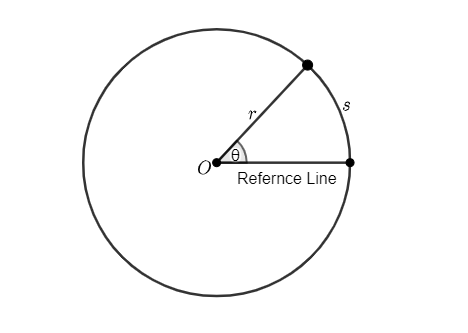Homework help for Dynamics of Rotational Motion

# Answers for dynamics of Rotational Motion problems

Physics homework helps for all questions on the effect of force, mass, moment of inertia & more!

## What is Rotational Dynamics?

Rotational dynamics refers to the study of rotational motion of a body along axes, under the action of torque.

### Angular Position and Angular Displacement

To measure the angular position, we need a reference line fixed in the body also rotating with the body, and perpendicular to axes of rotation.In the above diagram, point O is the axes of rotation. And,is the angular position, which is measured in radians. And, r is the radius.Angular displacement is the measure of the angle in radian, by which the position of point changed.is the angular displacement between positionand### Average Angular Velocity

Average angular velocityof the body with angular displacementduring the time intervalwould be:### Angular Acceleration

Angular acceleration could be calculated by taking the ratio of change in angular velocityand time interval.### Equation of Rotational Motion

The following equations are true for the constant acceleration.## Motion Questions & Answers – Sample

Question 1: Calculate the angular displacement of a student running on a circular field, with a radius of 35 m, and the student has covered a 50 m distance from his starting point.

Explanation: The radius of curvature is 35 m, and the distance traveled is 50 m.

So,And, we have,Final answer: The angular displacement would beQuestion 2: Calculate the angular velocity of a rotating body about an axis after, having a constant angular acceleration of. Initially, the object was at rest.

Explanation: We will use the equation of motionFinal answer: The angular velocity would beQuestion 3: Calculate the angular acceleration of a body, which gains an angular velocity of 63 rad/s, during the first 8 seconds.

Explanation: The body was initially at rest. So,, andAlso, after,Final answer: The angular acceleration would be.

## Round-the-clock help with rotational dynamics practice problems, formulas and equations for better grades

Rotational dynamics can be defined as angular displacement of an object in motion or refers to an object that is moving in a curved path. It involves study of rotational inertia, torque, angular velocity and angular displacement.

High schoolers or College students can take 24/7 assistance from our qualified experts to understand how to use vector quantities for describing the kinematics of rotations and solve complex questions.

Whether you need to solve exercises at the end of the Physics chapter on rotational dynamics or need clarity on some equations given on a worksheet- we’re here to help you find solutions.

Let’s help you understand how objects rotate or assist you to deal with analyzing the general relationship between torque, angular acceleration and moment of inertia. All in all, our top experts are your best bet!

Our assignment help services are designed to help you or VARK (Visual. Auditory, Read/Write and Kinesthetic) learners figure out how to describe objects that resist rotational motions.

With the help of our top aides get help with formulas, equations and regular practice problems in the subject.

## Take these few simples steps to get rotational dynamics Physics help

To get top quality assignment with zero plagiarism issues just follow few simple steps to get timely help:

Step 1: Fill out our quick order homework help form with detailed instructions for the expert

Step 2: Hire a Physics solver who agrees to your proposed budget and given timeline

Step 3: On receiving your assignment draft, quickly review before releasing payment from escrow

Our experts churn out 100% original and best quality reports with the sole aim to help you gain clarity on tough Physics concepts (Internal link to the physics homework help page). Moreover our Money Refund policy is here to cement your trust and satisfaction in availing our homework help services.

So get ready to meet deadlines by availing top assistance in calculating net torque or “which cylinder will reach the bottom of the incline first?” by getting guidance and step-by-step explanations to simplify your curriculum.

### What are rotational dynamics?

Rotational dynamics refers to the study of the rotational motion of a body along axes, under the action of torque.

### How to solve rotational dynamics problems?

To solve the rotational dynamics problem, we need an understanding of the equation of rotational motion.

Equation of rotational motion:Some other important formulas:,,Where angular velocity of the bodywith angular displacementduring the time interval, and with angular acceleration α.

### What is an example of rotational motion?

The motion of the Ferris Wheel, the motion of the motor, rotational of the earth around its axes are some examples of rotational motion.

### What are the important characteristics of rotational motion?

The rigid body undergoes a circular motion about an axis perpendicular to the plane and the center of the circular path lies on the same axes.

### Is circular motion and rotational motion the same?

In a circular motion, the particle moves around a circular path. For example, the moon is revolving in a circular path.

However, in rotational motion, the particles move in a circular path around axes perpendicular to the plane. For example, the Ferris Wheel is rotating around its axes.

So, we can say, the circular motion and rotational motion are not exactly the same.

### What is the difference between rotation and revolution?

In rotation, the particle moves around its own axes which is perpendicular to the plane. Example: motion of the fan.

And, in revolution, the object moves in a circular path around another object. Example: Earth revolving around the Sun.